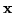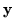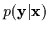Next: Machine Learning: Practical Considerations Up: Action-Reaction Learning: Analysis and Previous: Probabilistic Time Series Modeling

# Learning: Conditional vs. Joint

Beyond EM: Generalized Bound Maximization,
Conditional Densities and the CEM Algorithm

In the following chapters, we present a section dedicated to the machine learning aspect of this work. The concept of learning and probabilistic estimation is central to the Action-Reaction Learning paradigm and framework. The objective is to learn a mapping between the past interaction () and the most likely reaction () of humans from video data. This machine learning problem requires special attention and we describe a novel approach to resolving it. The contribution here is to stress that Action-Reaction Learning is best considered as a discriminatory learning problem and we show a formal Bayesian description of it. Essentially, we would like to estimate and use a conditional probability,. However, traditional tools such as the EM (Expectation Maximization)  algorithm do not apply. Therefore, we instead propose an optimization framework and derive the CEM (Conditional Expectation Maximization) algorithm to resolve the problem.

We discuss important differences between conditional density estimation and conditioned joint density estimation in practical machine learning systems and at a Bayesian level. This motivates the use of direct conditional estimation and maximum conditional likelihood. To this end, we introduce and argue favorably for the generalized bound maximization (GBM) framework. The approach encompasses a variety of bounding methods that are useful for many optimization and learning problems. In particular, we apply these techniques to develop the Conditional Expectation Maximization (CEM) algorithm which maximizes conditional likelihood. The GBM techniques extend the bounds computed by the EM algorithm and offer reliable optimization and convergence. In addition, the bounding techniques are more general and can be applied to functions which can not be posed as maximum-likelihood incomplete-data problems. Essentially, we reformulate gradient ascent steps as optimization of a bound and avoid ad hoc estimation of step size. The approach is guaranteed to maximize the given function at each step and local convergence is proven. Unlike gradient techniques, the GBM framework is amenable to deterministic annealing and can be used to search for global optima. Results are shown for conditional density estimation as well as nonlinear function optimization.Next: Machine Learning: Practical Considerations Up: Action-Reaction Learning: Analysis and Previous: Probabilistic Time Series Modeling
Tony Jebara
1999-09-15